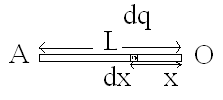# A charge Q is uniformly distributed over a long rod AB of length L . The electric potential at the point O lying at a distance L from the end A is: solve it by using the relation v=integration E.dr

9 years agoLet we take a small element of dq charge of dx length at a distance ''x'' from point O.  and then we will integrate it for potential of rod!

Thus, potential on a charge dq will be:

dV = Kdq/x = (kQ/l).dx/x

Integerating both sides from limits l to 0we get -

V = k(Q/l) [logl]

thus potential at point O comes  a constant value which is equals to:

V = k(Q/l) [logl].

Plz approve!